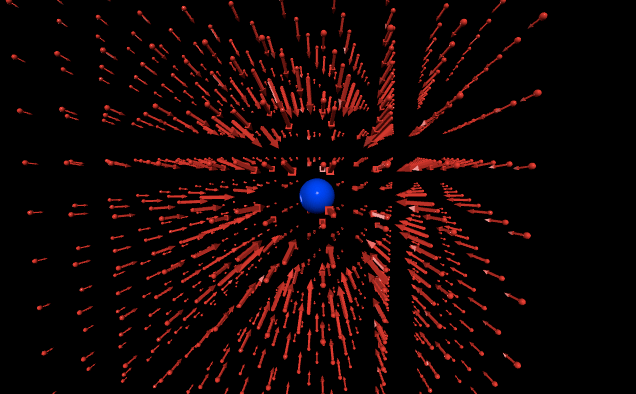# Day 44: Bridge from Coulomb’s Law Computational Model to Electric Fields

Today, we started class with students sharing their computational models. I then used one of the students models that attached a vector for the net force to the point charge to illustrate how their model showed the direction and relative magnitude of the electric force at every point through which the point charge moved. I then asked what we could do if we wanted to know the direction and relative magnitude of the electric force at every point around a charged object. Once they said that we would need a lot more point charges, I showed them this model:While tomorrow’s lesson will formally introduce the concept of the electric field and its relationship to the electric force, I did use this model as a bridge by introducing the visual of electric field vectors. I also showed them another model with multiple charged objects to demonstrate that the electric field vectors represent a superposition of the electric force due to each charged particle:I tried to write this model in a fairly extensible manner to make it easy to model various configurations of charged objects.

##electrostatics ##representations ##tech ##glowscript